# The Divergence

The divergence of a vector fieldin rectangular coordinates is defined as the scalar product of the del operator and the function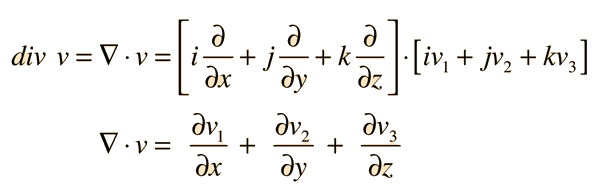The divergence is a scalar function of a vector field. The divergence theorem is an important mathematical tool in electricity and magnetism.

### Divergence in other coordinate systems

Index

Vector calculus

 HyperPhysics*****HyperMath*****Calculus R Nave
Go Back

# Applications of Divergence

The divergence of a vector field is proportional to the density of point sources of the field. In Gauss' law for the electric field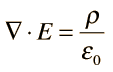the divergence gives the density of point charges. In Gauss' law for the magnetic field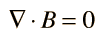the zero value for the divergence implies that there are no point sources of magnetic field.
Index

Vector calculus

 HyperPhysics*****HyperMath*****Calculus R Nave
Go Back

# Divergence, Various Coordinates

Compared to the divergence in rectangular coordinates: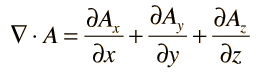In cylindrical polar coordinates: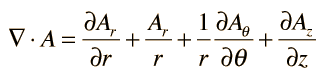and in spherical polar coordinates: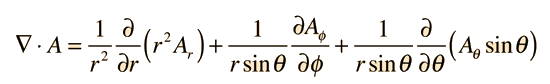Index

Vector calculus

 HyperPhysics*****HyperMath*****Calculus R Nave
Go Back